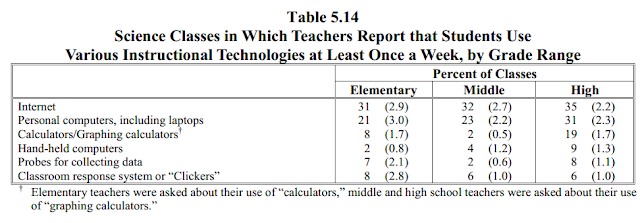### Calculators in Classrooms

In a previous post in this blog, "Why So Many Elementary Students Aren’t Mastering Basic Math Facts", Lynne Diligent wrote:
...Unfortunately, I am now running across a number of 14-year-olds who are using calculators to add 5 + 3, or 7 + 6, or 9 + 2. What’s even worse, THEIR TEACHERS LET THEM!!!! I personally think calculators should just be thrown out until about Grade 11, or whenever math involving higher functions on calculators is started. Prior to that time, they shouldn’t be allowed in school at all....
In one exam I wrote for General Chemistry, I asked the students to provide the number of ways by which I can distribute 10000 balls between two containers with one container having 9997 and the other the remaining 3 balls. The mathematical equation that provides the answer to this question is the following:Here, P  is the number of ways for N balls in x boxes, in which the occupancy is: nA is the number of balls in box A, nB is the number of balls in box B.
The number of ways for the above case, 10000 balls, 9997 in one box and 3 in the other, is equal to (10000!)/(9997!)(3!). The problem some students encountered is that 10000! on a calculator gives an error:

The number is too large. Of course, if one does some preliminary steps, the answer can still be easily obtained: (10000!)/(9997!) is really 10000 X 9999 X 9998. This is equal to 99970020000. Dividing this by 3! or 6, one arrives at the final answer, 16661670000. Being extremely dependent on calculators can prevent resourcefulness. In science where most numbers are actually measured numbers, it also becomes difficult to appreciate the significance of each digit. Students easily report an answer such as 1.23456789 grams since calculators provide these many digits when the correct answer should be just 1.2 grams. In this particular case, the digits after 2 are meaningless since these are beyond the precision of the original measured numbers. On the other hand, if students are required to do arithmetic operations by hand, dropping insignificant digits is very useful since the tasks become less cumbersome.

The issue of using calculators in classrooms is expected to be resurrected, as reported by Eric Robelen in Education Week. With Common Core and the new assessments, the objective of uniformity is likewise an issue not just in the contents of the exams, but also in the mechanics or rules on how students answer these exams. Robelen notes:
...Policies emerging from the two state consortia developing common-core assessments would prohibit most students from using calculators on the grades 3-5 tests, for example. At grades 6 and above, they call for calculator "on" and "off" sections and set restrictions on what functionality is allowed. (Both consortia will provide online calculators for the computer-based tests.)....
Grades 3-5 tests are expected to gauge a student's ability to perform arithmetic operations so it is understandable why calculators are not allowed. At higher grades, the debate remains. For the Graduate Record Examination (GRE) in chemistry, a test taken by chemistry majors applying for graduate school, calculators are not allowed:
...Test questions are constructed to simplify mathematical manipulations. As a result, neither calculators nor tables of logarithms are needed. If the solution to a problem requires the use of logarithms, the necessary values are included with the question.... (http://www.ets.org/gre/subject/about/content/chemistry)
How often are calculators used in US K-12 schools? The 2012 National Survey of Science and Mathematics Education reports the following:Above table copied from National Survey of Science and Mathematics Education
The calculator is, without any doubt, a very useful tool. What needs to be emphasized is when one should use it, and when one should not use it. It is about appropriateness. It is in fact the same question one should ask when deciding to use any tool technology offers inside the classroom.